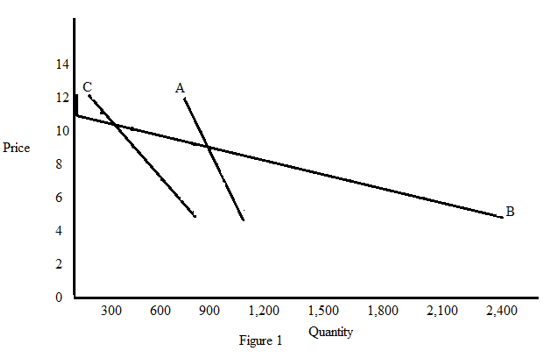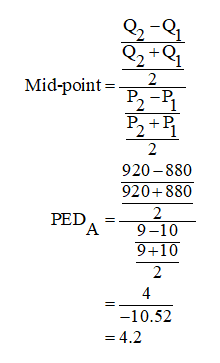# A local firm produces three types of Pizza, for delivery to homes in the area. The owner has completed research, to discover the demand curves for each of the three pizzas. The schedules are shown below: (Quantities are per week).  PricePizza A (Qd)Pizza B (Qd)Pizza C (Qd)12800010011840020010880400300992080040089601200500710001600600610402000700510802400800 Plot the three demand curves, on one graph. (It does not have to be Excel). Calculate PED for all three pizzas over the price range \$9 to \$10.  Use the midpoint formula (see your class lecture notes.) Explain why the three PED measures are different.  What change would make all three PED measures become more elastic?  What about more inelastic?

Question
23 views

A local firm produces three types of Pizza, for delivery to homes in the area. The owner has completed research, to discover the demand curves for each of the three pizzas. The schedules are shown below: (Quantities are per week).

 Price Pizza A (Qd) Pizza B (Qd) Pizza C (Qd) 12 800 0 100 11 840 0 200 10 880 400 300 9 920 800 400 8 960 1200 500 7 1000 1600 600 6 1040 2000 700 5 1080 2400 800

Plot the three demand curves, on one graph. (It does not have to be Excel).

1. Calculate PED for all three pizzas over the price range \$9 to \$10.  Use the midpoint formula (see your class lecture notes.)

1. Explain why the three PED measures are different.  What change would make all three PED measures become more elastic?  What about more inelastic?

check_circle

star
star
star
star
star
1 Rating
Step 1

Price elasticity of demand refers to the degree of responsiveness of demand for a commodity, after a change in its price. The percentage method, mid-point method, and the arc method are the three important measures of price elasticity of demand.

Step 2

The demand curves for all the three pizzas can graphically be explained as follows.Figure 1 represents the demsnd curves of pizza A, B and C. In this figure, price is measured on the vertical axis and the quantity demand is measured on the horizontl axis. The demand curve for pizza A is more steeper and the demand curve foe pizza 'B' is more flatter.

Step 3

The price elasticity of demand for all the three pizza's over the price ranges of \$9 to \$10 can be calculated by using the mid-point method as follows.The calculated value shows that the price elasticity of demand for pizza A over the price ranges of ...

### Want to see the full answer?

See Solution

#### Want to see this answer and more?

Solutions are written by subject experts who are available 24/7. Questions are typically answered within 1 hour.*

See Solution
*Response times may vary by subject and question.
Tagged in